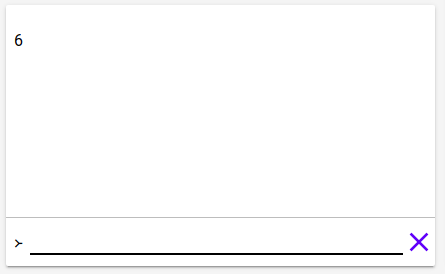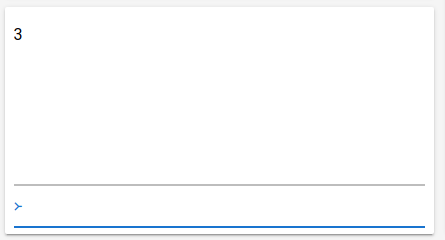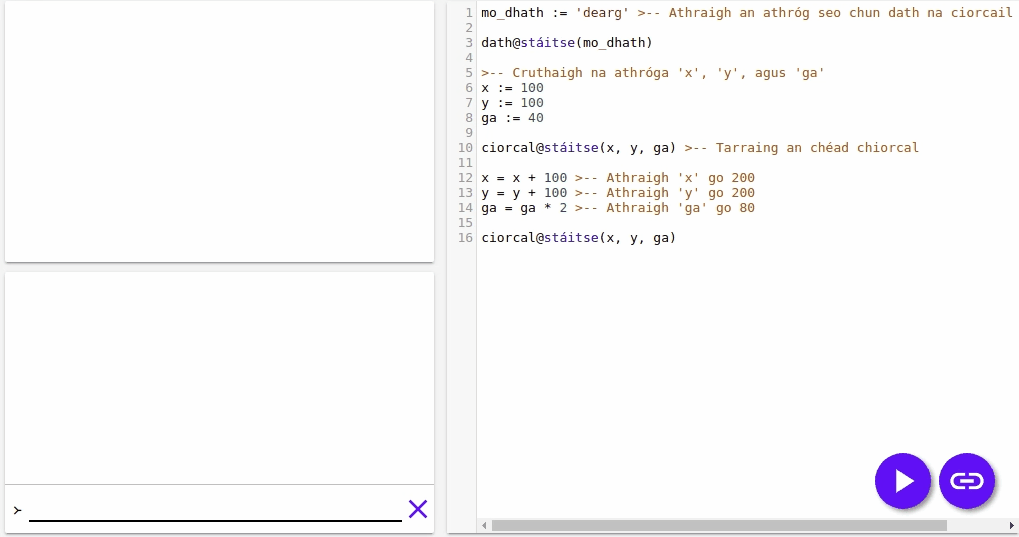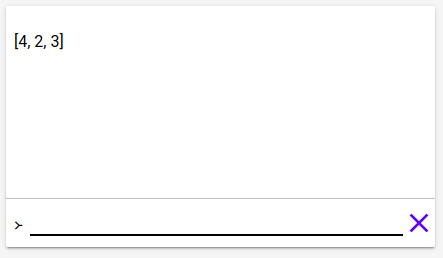# Setanta 101

## Maths

Setanta can do maths. Type `scríobh(2*3)` into the editor, click start, and look at the console. `6` will be written there.Look what this program writes.

``````scríobh(2 + 2)
scríobh(4 * (2 + 3))
scríobh(5 == 5)
scríobh(5 == 6)
scríobh(5 != 6)``````

This is in the console.

``````4
20
fíor
bréag
fíor``````

Specifically, notice that `==` and `!=` compare numbers (and other things).

## Text

You can write text in your programs too, we saw this earlier with the simple program:

``scríobh('Dia duit')``

You create text with two `'`s. For example:

``````'Hello'
'My name is Cú Chulainn'``````

You can use `+` to join two text together.

``scríobh('Hello' + ', ' + 'my name is Eoin')``

This writes `Hello, My name is Eoin`.

## Lists

Create lists in Setanta with `[` and `]`, for example:

``````[1, 2, 3]

Lists are very powerful. Every position in a list has a number. You can get an element of a list if you have the number of the position of the element. We give the number 0 to the first element of the list, the number 1 to the second element, the number 2 to the third etc.

If you have the number of the position, you can use `[` and `]` again to get the member. For example:

``````['Dia', 'Duit'] == 'Dia'
['Dia', 'Duit'] == 'Duit'

[100, 200, 300, 400] == 300``````

You can use `+` with two lists to make a new list:

``[1, 2, 3] + [4, 5, 6] == [1, 2, 3, 4, 5, 6]``

If you write the symbol `>--` in your program, Anything you write after it on the same line is a comment, and is not part of the program. For example

``````scríobh('Hello') >-- I can write anything here!
>-- Or here as well``````

Comments are very useful because they allow us to write notes in our programs.

## Variables

You use variables to store numbers, lists or anything. You put something in the variables and later you can get it back. Try this out for example:

``````x := 10
scríobh(x * 2)``````

It writes out `20` in the console. The first line put `10` in `x` and the second line used it.

In this program `x` is a variable. We can use variables everywhere that we use numbers, text or anything.

We create new variables with `:=`.

We can change the value in the variable `x` with `=`.

``````x := 10 >-- New variable with value 10
x = 20``````

Now `x` is equal to 20.

When you use `=` or `:=`, Setanta computes the term on the right, and then puts the result in the variable on the left. Because of this, we can use the same variables on the left and the right. For example:

``````x := 1
x = x + 2
scríobh(x)``````What does this program write?

``````x := 10
y := 2 * x >-- Create a new variable 'y' with value 20
x = y + x >-- Change the variable 'x' to 'x + y' = 10 + 20 = 30
scríobh(x)``````

We can use variables to draw things on the stage too. Put the following code in the editor:

``````mo_dhath := 'dearg' >-- Change this variable to change the colour of the circles.

dath@stáitse(mo_dhath)

>-- Create the variables 'x', 'y' and 'ga'
x := 100
y := 100
ga := 40

ciorcal@stáitse(x, y, ga) >-- Draw the first circle

x = x + 100 >-- Change 'x' to 200
y = y + 100 >-- Change 'y' to 200
ga = ga * 2 >-- Change 'ga' to 80

ciorcal@stáitse(x, y, ga)``````## Changing lists

We can change members of lists with `=` too. For example:

``````l := [1, 2, 3]
l = 4
scríobh(l)``````The first value of the list has changed!

## Next

You learned a lot of new things, go to the next page to learn about `má` and loops.